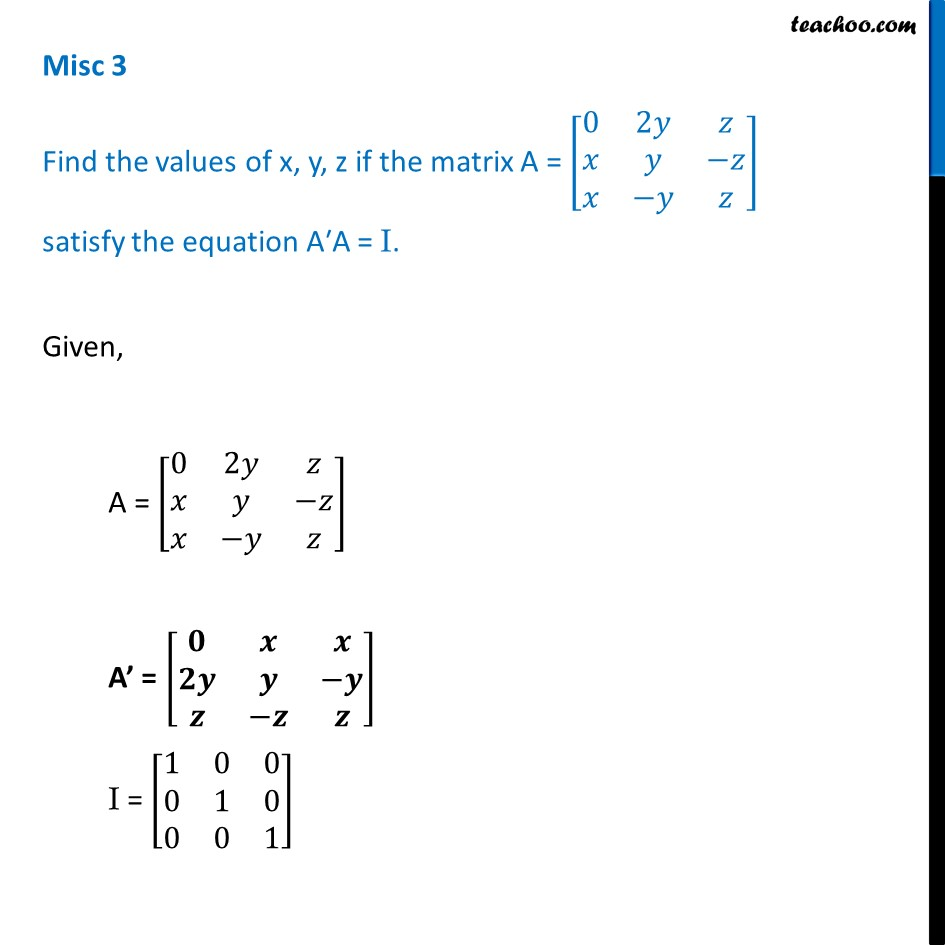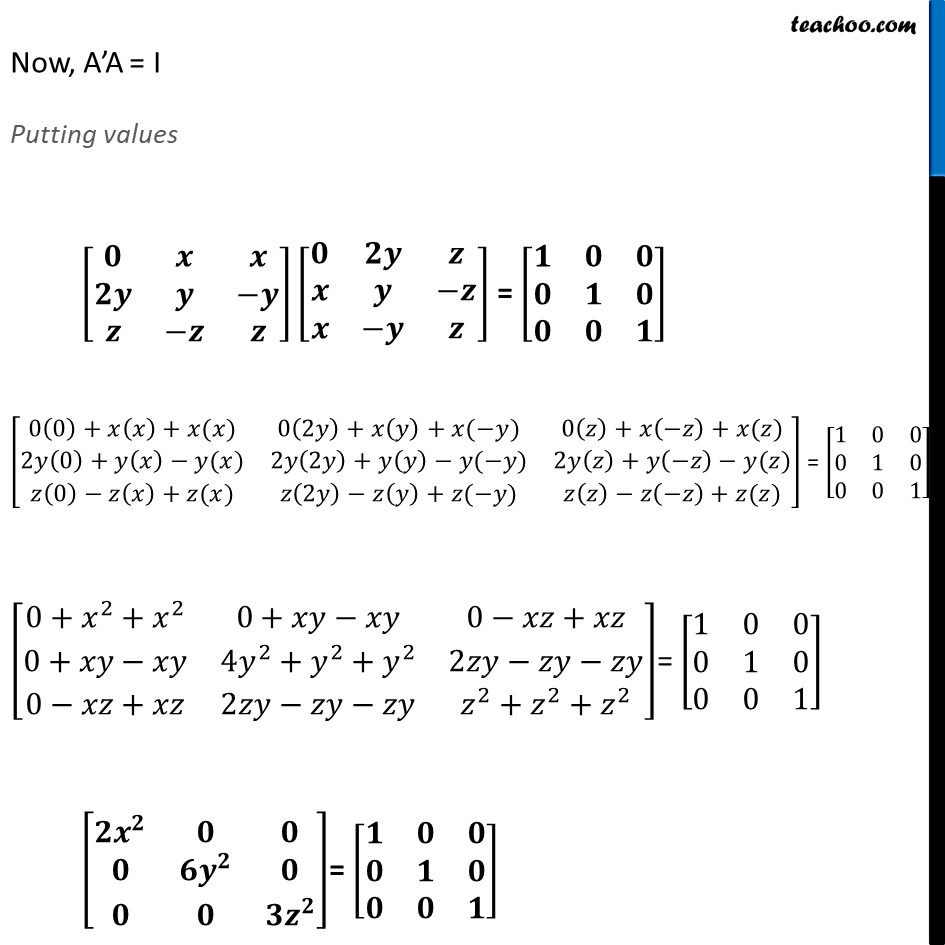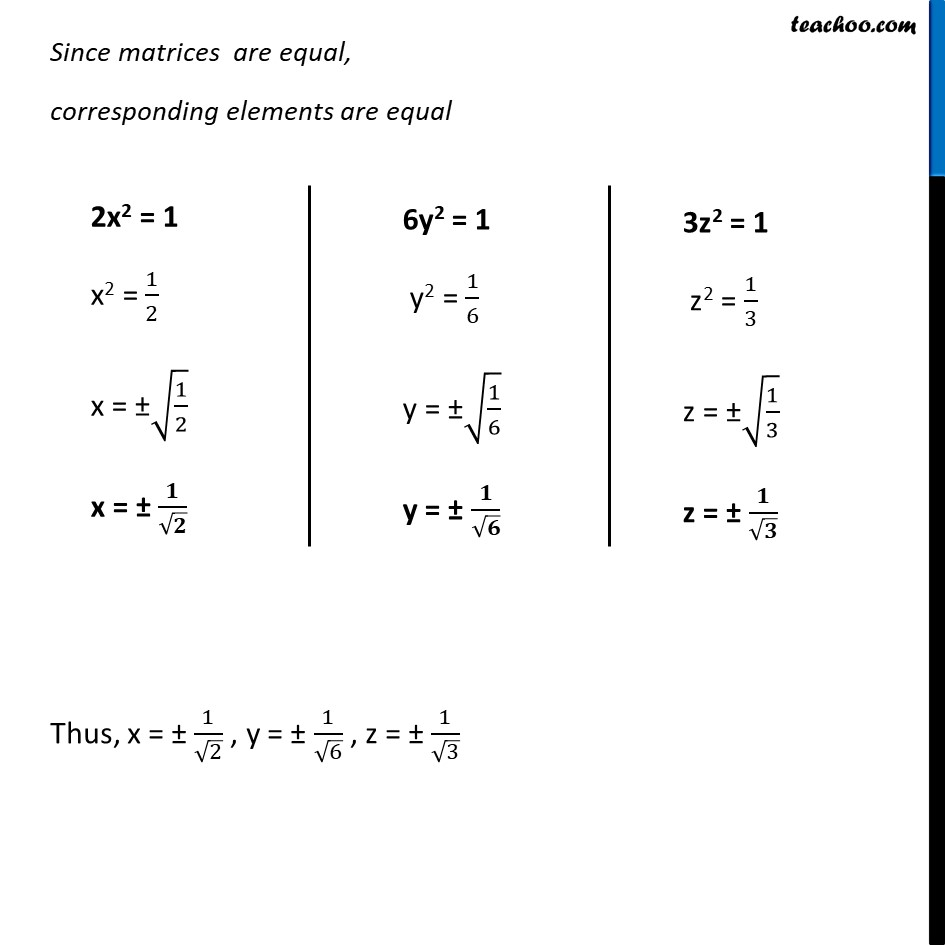Miscellaneous

Chapter 3 Class 12 Matrices
Serial order wiseLearn in your speed, with individual attention - Teachoo Maths 1-on-1 Class

### Transcript

Misc 3 Find the values of x, y, z if the matrix A = [■8(0&2𝑦&𝑧@𝑥&𝑦&−𝑧@𝑥&−𝑦&𝑧)] satisfy the equation A′A = I. Given, A = [■8(0&2𝑦&𝑧@𝑥&𝑦&−𝑧@𝑥&−𝑦&𝑧)] A’ = [■8(𝟎&𝒙&𝒙@𝟐𝒚&𝒚&−𝒚@𝒛&−𝒛&𝒛)] I = [■8(1&0&0@0&1&0@0&0&1)] Now, A’A = I Putting values [■8(𝟎&𝒙&𝒙@𝟐𝒚&𝒚&−𝒚@𝒛&−𝒛&𝒛)][■8(𝟎&𝟐𝒚&𝒛@𝒙&𝒚&−𝒛@𝒙&−𝒚&𝒛)] = [■8(𝟏&𝟎&𝟎@𝟎&𝟏&𝟎@𝟎&𝟎&𝟏)] [■8(0(0)+𝑥(𝑥)+𝑥(𝑥)&0(2𝑦)+𝑥(𝑦)+𝑥(−𝑦)&0(𝑧)+𝑥(−𝑧)+𝑥(𝑧)@2𝑦(0)+𝑦(𝑥)−𝑦(𝑥)&2𝑦(2𝑦)+𝑦(𝑦)−𝑦(−𝑦)&2𝑦(𝑧)+𝑦(−𝑧)−𝑦(𝑧)@𝑧(0)−𝑧(𝑥)+𝑧(𝑥)&𝑧(2𝑦)−𝑧(𝑦)+𝑧(−𝑦)&𝑧(𝑧)−𝑧(−𝑧)+𝑧(𝑧))] = [■8(1&0&0@0&1&0@0&0&1)] [■8(0+𝑥^2+𝑥^2&0+𝑥𝑦−𝑥𝑦&0−𝑥𝑧+𝑥𝑧@0+𝑥𝑦−𝑥𝑦&4𝑦^2+𝑦^2+𝑦^2&2𝑧𝑦−𝑧𝑦−𝑧𝑦@0−𝑥𝑧+𝑥𝑧&2𝑧𝑦−𝑧𝑦−𝑧𝑦&𝑧^2+𝑧^2+𝑧^2 )]= [■8(1&0&0@0&1&0@0&0&1)] [■8(𝟐𝒙^𝟐&𝟎&𝟎@𝟎&𝟔𝒚^𝟐&𝟎@𝟎&𝟎&𝟑𝒛^𝟐 )]= [■8(𝟏&𝟎&𝟎@𝟎&𝟏&𝟎@𝟎&𝟎&𝟏)] Since matrices are equal, corresponding elements are equal Thus, x = ± 1/√2 , y = ± 1/√6 , z = ± 1/√3 2x2 = 1 x2 = 1/2 x = ±√(1/2) x = ± 𝟏/√𝟐 6y2 = 1 y2 = 1/6 y = ±√(1/6) y = ± 𝟏/√𝟔 3z2 = 1 z2 = 1/3 z = ±√(1/3) z = ± 𝟏/√𝟑